# COUNTIF Function

It is an inbuilt function in Excel that counts the cell in a range that meets specific criteria.Reviewed by

Osman Ahmed

Expertise: Investment Banking | Private Equity

Updated:

August 29, 2023

## What is the COUNTIF Function?

The COUNTIF function is an inbuilt function in Excel that counts the cell in a range that meets specific criteria.

Big data has revolutionized companies worldwide. Even though Excel might not be best suited to handle such vast volumes of data, it makes it easier with the number of data analysis functions the software offers, one of which is the function.

Excel offers different functions that perform a similar task, such as COUNTBLANK, which counts the number of blank cells; COUNTA, which counts all the cells in the range; and COUNTIFS which counts cells based on multiple criteria.

The function uses different logical operators to input conditions which, if returned as TRUE for a particular cell, will lead to an increase in the count for the final result.

Similarly, the function also allows wildcard characters to make a partial match for a text string and, if found, leads to an increase in the count for the result.

This article will guide you in understanding the function's syntax and how you can use the process to count cells that meet mentioned conditions.

## Understanding The COUNTIF function

The function is categorized as a statistical function that counts the number of cells based on predetermined conditions. If the condition is met, i.e., if the function evaluates to TRUE, then the value is counted as 1, and so on for a range of cells.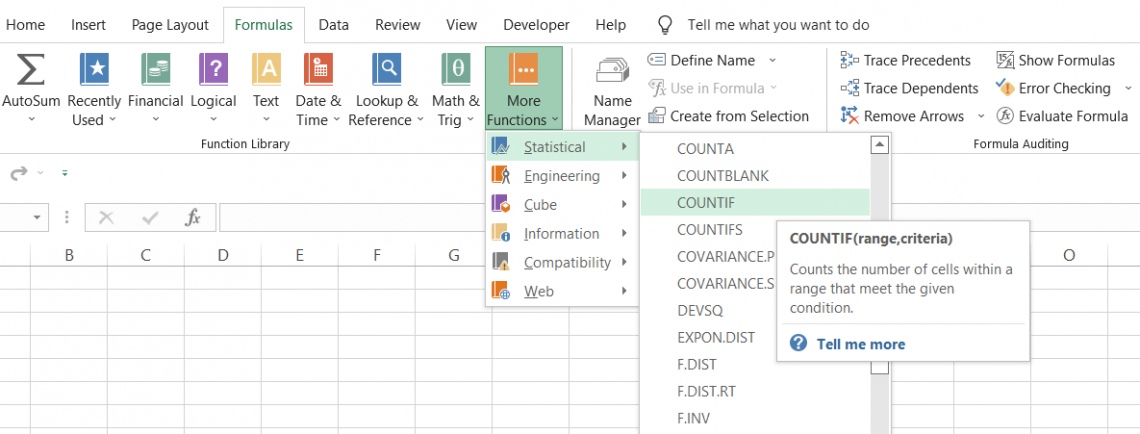Let's say you have ten cells, and only eight meet the specified criteria; the function returns the result as eight.

The logic behind the function is that it iterates through all the cells and only counts those cells which evaluate TRUE for the specified criterion.

The function can count cells containing dates, numbers, text strings, and blank cells.

The syntax for the function:

The syntax for the function is

=COUNTIF(range, criteria)

range - (required) the referenced field of cells that will be counted for the specified criterion

criteria - (required) the condition that must be met to count the cells

Now that we know the function, it is time to understand its two utmost important components - logical operators and wildcards.

a) Logical operators

The logical operators play an essential role in specifying different criteria in the function. In addition, these comparison operators help compare numbers and strings and perform complex assessments of the conditions.

Listed below are the logical operators you can use while using the function.

Logical Operators
ConditionOperatorDescription
Equal to =Compares two values and returns TRUE if they are equal,
Not equal to <>Compares two values and returns TRUE if they are not equal
Greater than>If one value is greater than the other, then the condition evaluates to TRUE
Less than<If one value is less than the other, then the condition evaluates to TRUE
Greater than or equal to >=The condition returns as TRUE if the value is greater than or equal to another value
Less than or equal to <=The condition returns as TRUE if the value is less than or equal to another value

Even though logical operators can further improve the efficiency of using the function, it is not essential to include them in the criteria. It is entirely up to you whether you use them or not.

b) Wildcard characters

Wildcards are special characters that return results by substituting them with other characters.

Including wildcard characters is another way to take the number-crunching part to another level, but it isn't entirely mandatory.

There are several wildcard characters that you can use, each of which functions differently with a supplied text string. Some wildcard characters are:

Wildcard Characters For The Function
CharacterExplanationExample
*It matches the characters before * with text and returns all the strings. he* will return hello, hell, hebrew but not chef, chew. It would have returned the latter words for che*
?It will match a single alphabet position of a text string.bee? Will return beer, beep, beet, etc
[]It will match characters within the square bracketsbee[rt] will return beer, beet but not been
[ ] + !An exclamation mark followed by characters inside brackets excludes them from the text matchbee[!rt] will not return beer, beet but will return beep, been
[ ] + -A range of alphabets(in ascending order) separated by a dash in brackets matches all the similar text in the string a[a-c]c will return aac, abc, acc
#It will match numerical characters.2#2 will return 252, 212, 222Begin your journey into Excel modeling with our free Excel Modeling Crash Course.

## How to use COUNTIF Function?

There are two different ways you can use the function. In this section, we will explore both methods so you can decide what method suits you the best.

a) Method #1: From the function's library,

Functions are the predetermined formulas where you need to input the arguments and directly get the result in the selected cell. For example, to use the function, please follow the steps below:

1. Select the cell in which you need the result for cell count based on the criteria.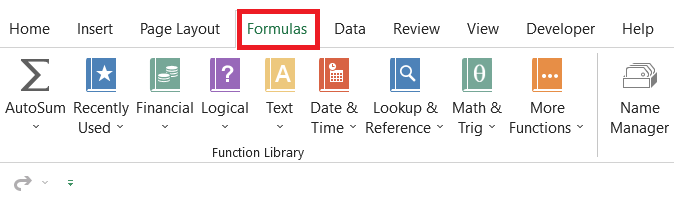2. Click on the Formulas tab > More Functions > Statistical, search for the function, and click on it.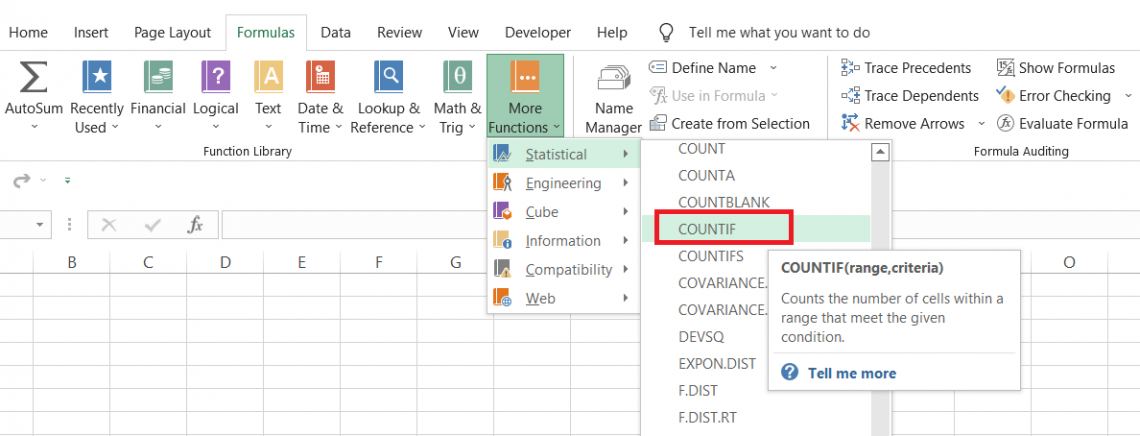3.  This will open up the dialog box as illustrated below: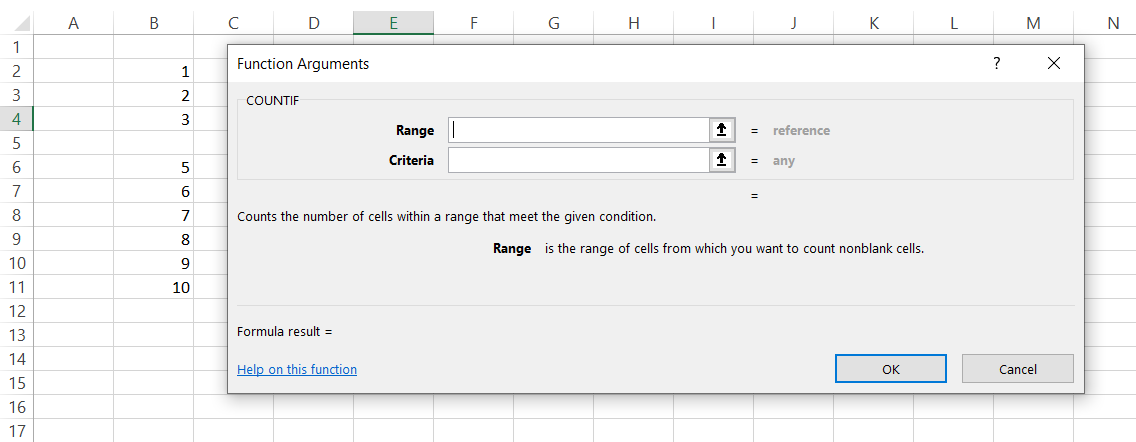4. We will try to find how many blank cells we have in the referenced range. For this, the 'range' and 'criteria' will be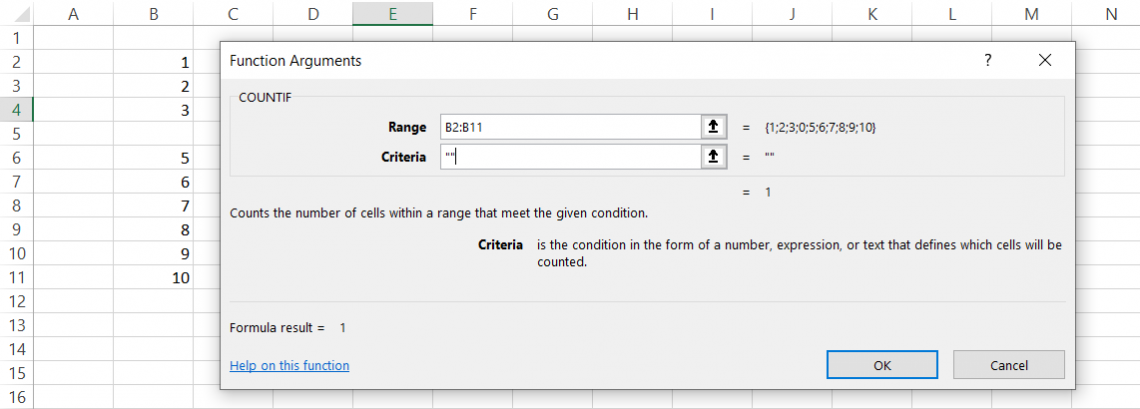5. As you can see, the function previews the result below the two arguments, equal to 1. This means we only have one cell in the range that is empty.
6. When you press Enter, the result in the selected cell is 1.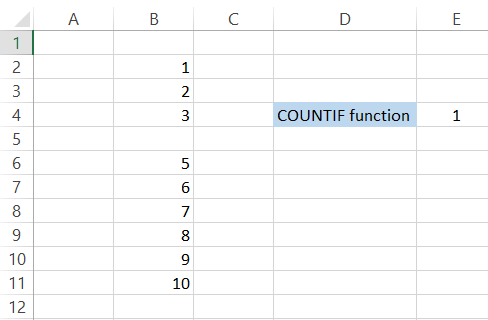b) Method #2: As a worksheet function

To use the function as a worksheet formula, you begin with the equal sign and input the formula name followed by the arguments in the function.

Suppose you need to count how many instances the word 'Excel' exist in the range of cells: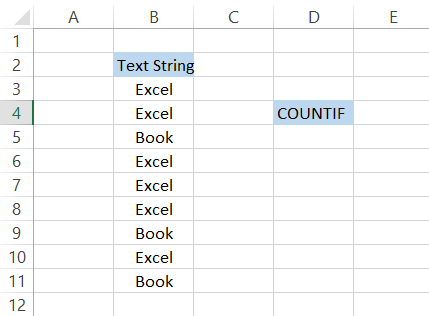Our range is B3:B11 while the condition is "Excel." Therefore, we will input both arguments such that the formula in cell E4 becomes

=COUNTIF(B3:B11, "Excel"),

Which will give us the result: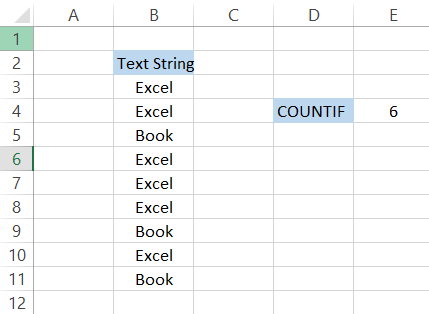Only on six instances do our criteria match the range of the cells, and thus we get the result as 6.### Everything You Need To Master Excel Modeling

To Help You Thrive in the Most Prestigious Jobs on Wall Street.

## COUNTIF Function Example

There are numerous occasions where the function can make your life much easier.

From reconciliations to data analysis, the function supports all the arithmetic calculations to make life easier for the Excel user. For example, you can count the numbers in data and the 'n' number of text strings.

Once you get the result based on the predefined condition, you can then go ahead and conduct further analysis of the dataset and make necessary interpretations.

It also accepts wildcard characters and is available in almost all versions of Excel, which is why using the function is a no-brainer.

In the consecutive sections, we will see some examples to help us understand the function better.

### Example #1 - Counting based on text strings

Suppose you either day trade for a living or work at a prominent institutional investor. First, you need to determine how many businesses you took for a particular stock from the trade book. The data looks as illustrated below: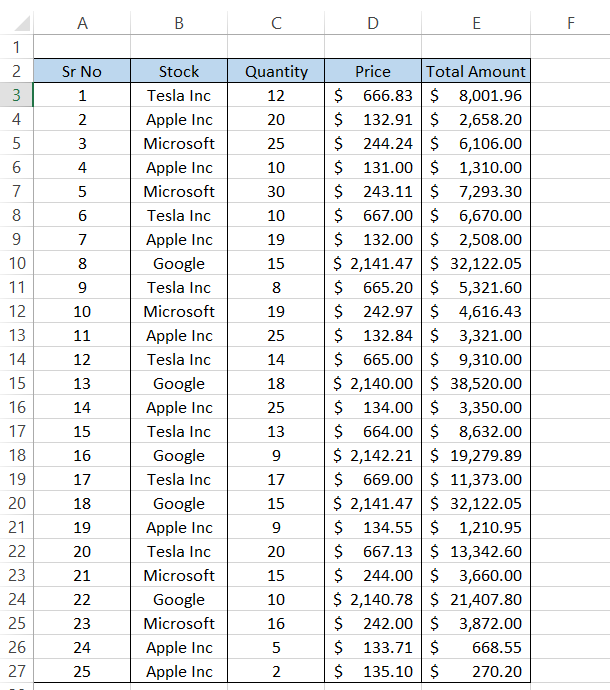To find the trade count for 'Tesla Inc,' we will use the formula =COUNTIF(B3:B27, G5) in cell H5, giving you the result as 7.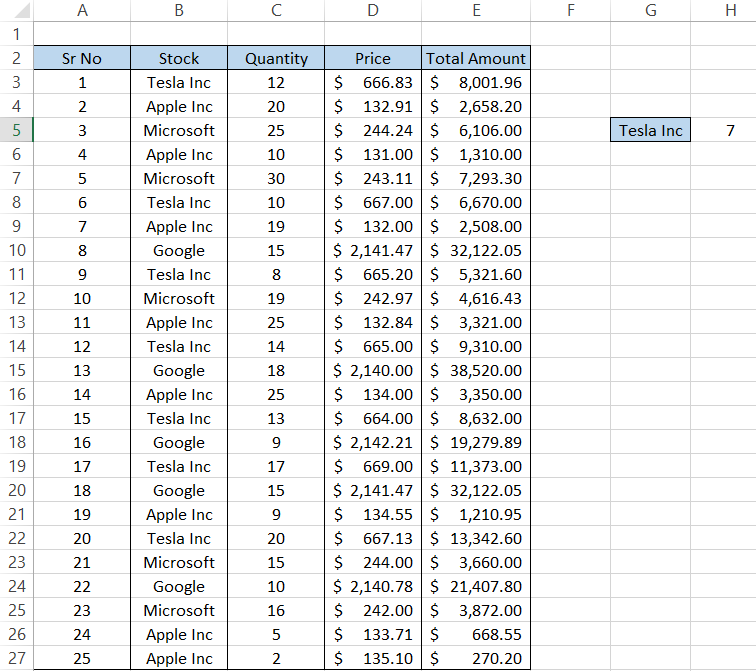We know 'Tesla Inc' exist in our data set since it occupies the first row in our dataset. But apart from that, what else do we have? And the question is - how do we find the different uniques?

The easiest trick in finding the unique is selecting the data and adding a filter to the data using the keyboard shortcut Alt + H + S + F.

Next, go to the column header 'Stock' and check the drop-down. You will get all the unique as: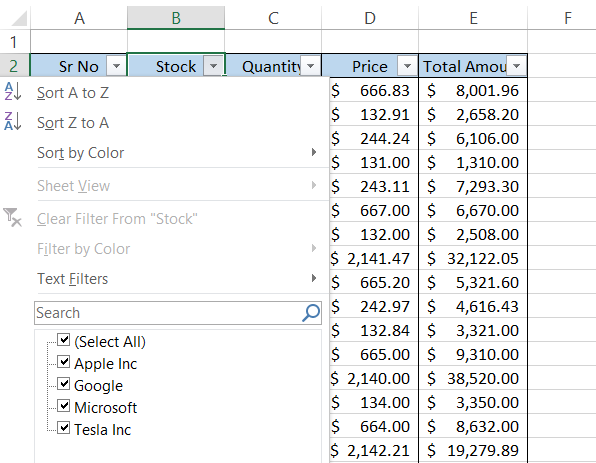Now, we can substitute them in the formula and find their respective count too!

We had 25 rows of data in our table. After substituting the 'criteria' into our function, we will get the result as illustrated below: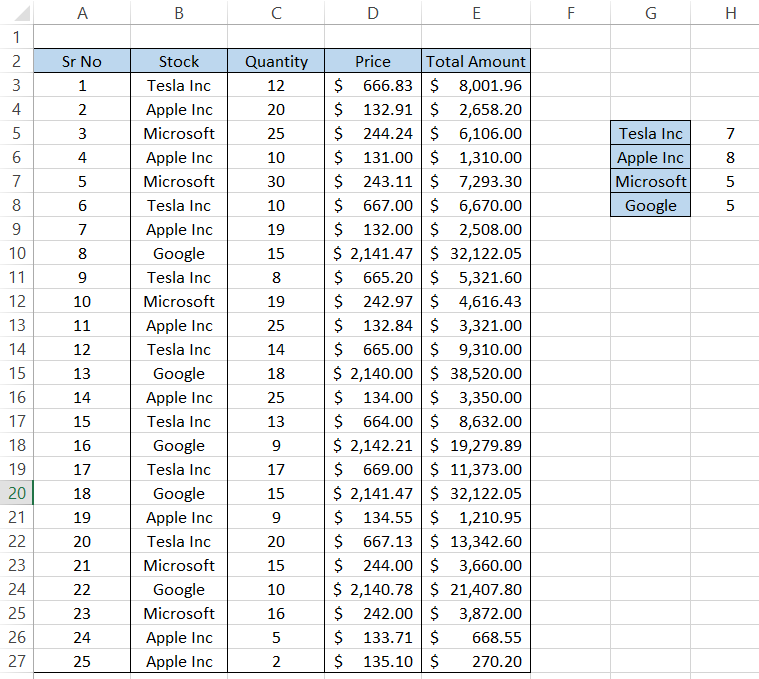Just input the text strings(stocks) in G6:G8 and drag the formula in H5 down to the H8 cell, and you will get the expected counts.

### Example #2 - Counting empty cells

Empty cells can be a big nuisance for your data analysis excel models. You can't ignore them since they can skew the data distribution. Empty cells arise as a result of the failure of Excel to capture the data that should have otherwise existed in those cells.

Thus, you must know how many cells in the data are empty.

Suppose that you have the dataset as illustrated below: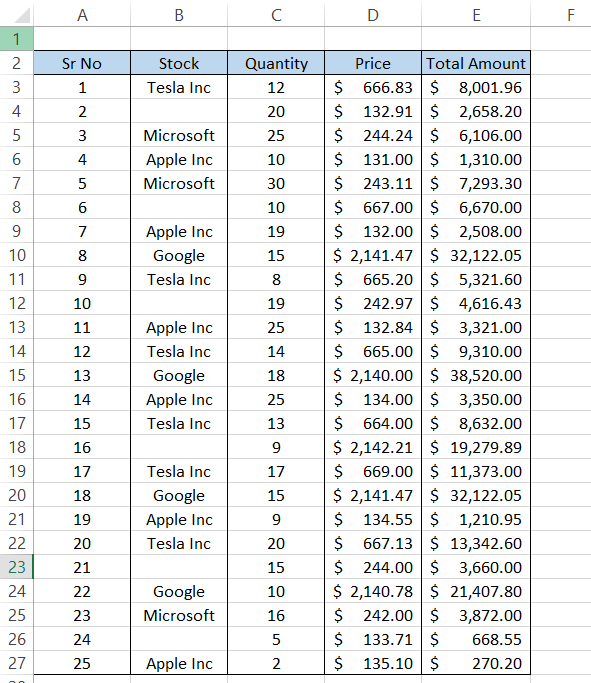Some of the cells in column B are empty. But to be precise - How many?

Here, we will use the formula =COUNTIF(B3:B27,"), giving us the result as 6. A total of six empty cells exist in column B.

Assessing the columns using this formula for empty cells before constructing dashboards and graphs can take your data analysis game to the next level.

Suppose that you find 78 non-empty numerical cells in a range of 80 cells. No problem. Almost 67 cells are empty in another column, while only 13 cells have a non-empty numerical value.

Does it make sense to include the latter column in our data analysis model? Not Really. Of course, you wouldn't be able to find this interpretation without the function quickly.

### Example #3 - Counting based on wildcards

Accounting ledgers usually have a substring that keeps repeating in the entire accounting if it corresponds to a particular party.

Let's say you intend to look for the number of times the transport expenses were paid for the entire month. The underlying assumption is that the vendor pays transport expenses greatly each month.

Suppose that the data looks as illustrated below: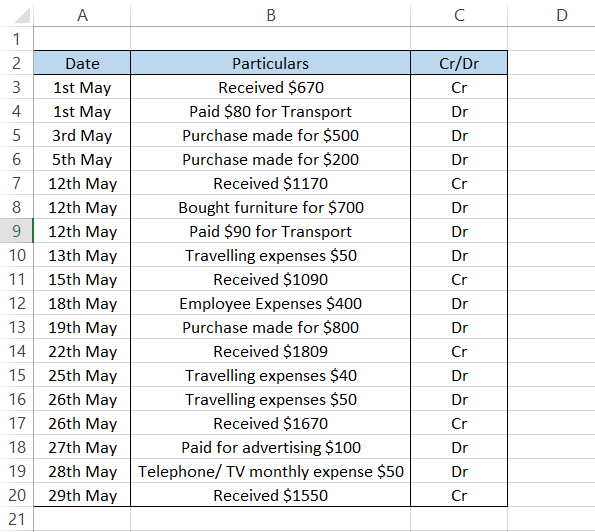We need to check whether all the payments for the transport were made or are still pending with us. On closer inspection, we know that our ledger's keyword 'transport' is standard.

You can use the formula

=COUNTIF(B3:B20, "*Transport*"),

which will capture any cell with the substring transport and give us the result as 2.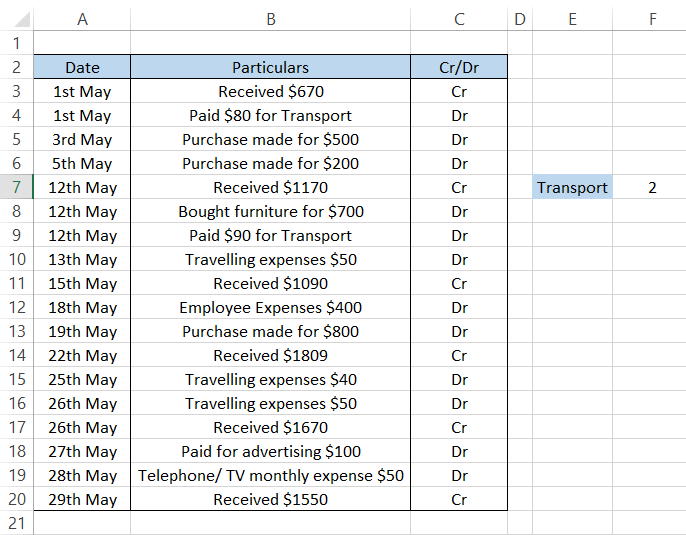Similarly, you can input different substrings and find the count of cells containing that specific substring.Begin your journey into Excel modeling with our free Excel Modeling Crash Course.

### Example #4 - Counting using logical operators

Suppose that your company made good sales for the day. The company starts operating at 8:00 AM and usually wraps up its business around 5:00 PM.

It would help to determine how many times the sales were less than $50. The data looks as illustrated below:To get the result, we will use =COUNTIF(D3:D12," <50"), Which will give us the effect as 2. By taking a close look, we can see that around 12:00 PM and 5:00 PM, we made$42 and \$41, respectively.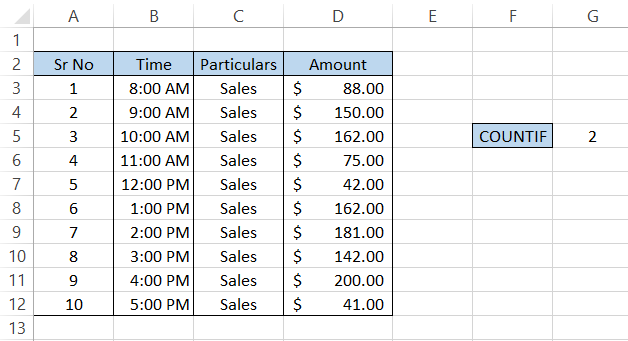You can also use a conditional formatting tool to highlight the cells matching the criteria.

Select the range of cells, click on the conditional formatting tool from the Home tab, and set a 'New Rule' from the drop-down. This will open up the dialog box where you will input the formula as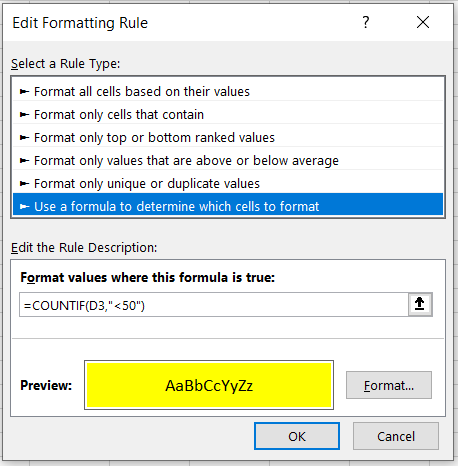Once you click on Ok, the formula will iterate through all the cells and highlight those that meet the specified criteria in the formula.

You will get the result as illustrated below: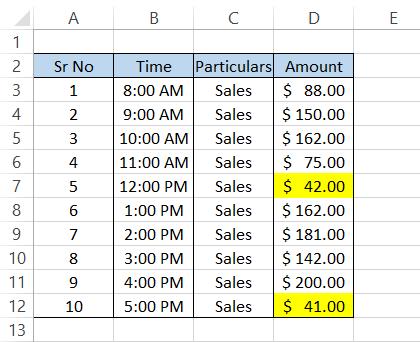We feel that the conditional formatting tool is a bit underrated for the range of abilities it provides to the user.

However, getting back to COUNTIF is another function that has limitless potential to take you to the next level in becoming an Excel wizard.

### Example #5 - Counting based on the value in different cells

Sometimes, you might get a date based on which you might need to evaluate how many dates in the data are more significant than or less than our 'base' date.

Yea, you could reference the date directly in the formula by nesting the DATE function, for example, =">" & DATE(2022,12,26), and the procedure should work file.

However, it's equally vital that you know how to reference a cell along with a comparison operator.

Suppose that you have the times as illustrated below: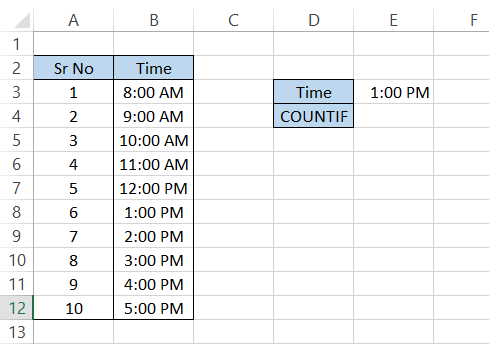First, we must find how many cells have a time greater than 1:00 PM. To get the result in cell E4, we will use the formula

=COUNTIF(B3:B12,">" &E3),

which will give us the effect as 4.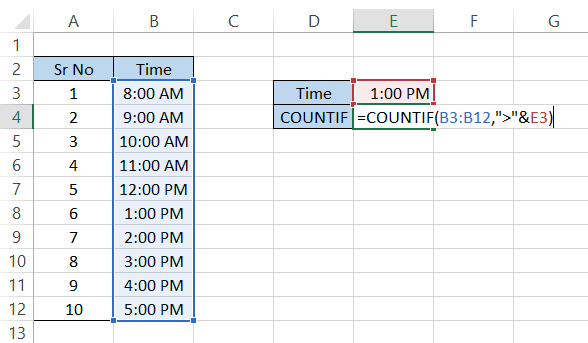As you are already aware, time also is represented in decimal numbers, where 0 is equal to 12:00 AM, and 1 is similar to 11:59:59 PM.

So by using the formula, we are checking what decimal numbers exist in the range that is greater than 1:00 PM or 0.54166666666, which gives the result as 4.

## Key Takeaways

• The COUNTIF function returns that count the number of cells based on predetermined conditions
• The function will not accept a text string longer than 255 characters for the 'criteria' argument.
• The function is not able to differentiate between 'TEXT' and 'text,' i.e., it does not return case-sensitive results
• If you are referencing text strings in the formula, you need to enclose it between the quotation marks("“)
• Using the wildcards enables you to make partial matches and then count the cells based on those partial matches.### Everything You Need To Master Excel Modeling

To Help You Thrive in the Most Prestigious Jobs on Wall Street.

Researched and Authored by Akash Bagul | Linkedin

Reviewed and edited by Parul Gupta | LinkedIn